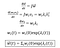# Deriving the conditions for Turing Patterns

Right before the unfortunate events that led to his untimely death, Alan Turing published a landmark paper “The Chemical Basis of Morphogenesis" that still deeply influences mathematical biology and in particular developmental biology. It describes how chemical patterns can be formed from diffusion processes; these chemical patterns in turn form a blueprint for the development of structures like the digits of our fingers and the stripes of a zebra.

The genius of Turing lies in that he saw that diffusion was not just a stabilising, homogenising force (since diffusion is the reason why a drop of ink spreads out and colour the whole glass of water uniformly) but it can also be the reason for instability resulting in patterns.

We start off by considering a 2 species reaction-diffusion system:

For Turing patterns, the instability has to be caused by the diffusion terms. Thus, we start off by considering the system without diffusion terms. This system without diffusion needs to be stable.

We perform linear stability analysis by first doing a Taylor expansion around the steady state (we assume that there is a steady state where the time derivatives du/dt = dv/dt =0).

Keeping only the first order terms, we obtain the following:

We then define a small perturbation w away from the steady state xs, :

The change in the perturbation over time is then:

We can also write this as:

For the steady state to be stable, w needs to tend to 0 as t tends to infinity.

Expressing w as the sum of its eigenfunctions,

We can then differentiate it ‘component’ by ‘component’:The differential of the eigenfunction is just itself multiplied by its corresponding eigenvalue

We then solve for the eigenvalues to determine if w tends to 0 over time. For the steady state to be stable, the real part of the eigenvalues need to be negative. Hence we obtain:

Here we see that for Re(λ)<0, tr(J)<0 and det(J)>0.

We can thus write the above condition as:

We now need to obtain the conditions for instability when there is diffusion. By repeating the above steps (taylor expansion of dx/dt, finding dw/dt and then rewriting it in matrix notation) we obtain:

Note that there is now an additional terms due to diffusion.

We now assume that there is a separable solution such that

The general solution for dw/dt is then:

Similar to the earlier section, we differentiate this expression and obtain:

For non-trivial solutions to exist, det(H - λI)=0. I.e. we are solving again eigenvalues. However, this time we want the real parts of the eigenvalues to be positive and thus, det(H) < 0.

We see that H depends on some constant k. Let’s solve for the minimum H for any k, since this is the necessary condition. To do that we take derivative of H over k.

We thus obtain the second condition for Turing patterns.